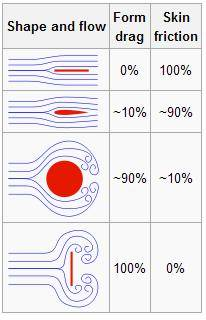# Calculating the Area considered to calculate Drag coefficient of a vehicle

• Ludomentis
In summary, the drag coefficient for a car is dependant on its orientation (facing upstream or downstream) and the Mach number. The drag coefficient for a half sphere depends on its orientation.f

#### Ludomentis

Hi ! First at all thanks for read, I'm new in the forum ...

So the problem is, I would calculate the drag coeff of a car but in the coeff formula I would like to know which AREA is considered for this.

drag coeff is given by = 2F / ρ(V^2) A

Where :
F is the drag force
ρ is the mass density of the fluid (air in this case)
V is the speed of the car
and A is the reference Area

is this that I can't understand. In Wikipedia (I know is not a good source) they say that this area is the projected area of the shape (vehicule) but if so... a sphere and a half sphere should have the same drag coeff. Or is not the case. It makes me doubt about this "orthogonal surface" calculation,

So this area is for exemple for a cube, side by side ? or for a sphere 4∏r^2 ? and for a half sphere 2∏r^2 ??

... and excuse my english I'm french

If you read the article carefully, you will note that the reference area is often NOT the surface area of the object. For the drag formula, the reference area of the sphere is the projected area normal to the flow, or pi * r^2, and this ref. area would be the same for a sphere or half-sphere with the same radius. The difference in the drag force is accounted for in the different drag coefficients Cd for the two shapes.

Ok but in the same conditions, what makes a half sphere have a lesser drag coeff than a sphere ?

The drag coefficient CD has a pressure and a viscous component.
As seen if the diagram. most of the drag for a sphere is due to form or pressure and a smaller contribution due to the skin friction.

The explanation that I can think of is as follows.
For a half sphere facing upstream, there is less surface area and subsequntly the percentage of surface friction is less, and that could expalin the lower value. Hopefully a fluid expert will verify.

Note that the drag coefficient for a half sphere depends on its orientation - whether it is facing into or downstream of the fluid flow.

Also, bear in mind that the coefficient is Reynolds number and Mach number dependant.
Calculate your R and M numbers so determine if the standard coefficient can be used. For a car the standard value should be OK.

A picture is always good.
http://gravity.wikia.com/index.php?title=Drag_(physics)&image=Flowsvg-jpgLast edited:
Thansk for answering, so it is impossible to calculate this Area (Front of the vehicule) by myself ? cars having a irregular shape, isn't ?

... and hte area considered is the area "touched" by the fluid, if I understood

In the Reynold Number

http://en.wikipedia.org/wiki/Reynolds_number

where:
is the mean velocity of the object relative to the fluid (SI units: m/s)
L is a characteristic linear dimension, (travelled length of the fluid; hydraulic diameter when dealing with river systems) (m)
μ is the dynamic viscosity of the fluid (Pa·s or N·s/m² or kg/(m·s))
ν is the kinematic viscosity (ν = μ / ρ) (m²/s)
is the density of the fluid (kg/m³)

The L cosidered is the same Area that I'm asking about ?

If you read Steamking's post, the reference area for CD is the projected frontal area, that is the area you would see if you looked at the object head on. Of course cars have a shape to them, so the CD for each car will be different. So the CD for a Volkwagon Jetta will be different for that of a Ford Mustang.

The CD includes, as mentioned already the skin friction and the pressure friction included thus as an all in one figure. One can thus look at the object and determine its drag force more readily than doing other but more complicated calculations and analysis.
A CD for a particulcar is obtained from wind tunnel tests I believe.

Here is a bunch of coefficients for selected normal day objects.
http://www.engineeringtoolbox.com/drag-coefficient-d_627.html

Notice that for cars they all say "frontal area".

As I mentioned before you use the Reynolds number ot the Mach number to determine whether or not the tabulated CD's are are applicable. Parameters for any equation, be it R, M, or CD do not vcarry over from one to the bext, so NO the L in Reynolds number has nothing to do with CD

Thanks I understood, thanls for answering and explain to me. In fact I'am in first year college and with this coeff and knowing the drag force formula, I could developp my first program applied to physics. Only giving analysis data, with several car coeff. (graphic of the Drag Force vs Speed...